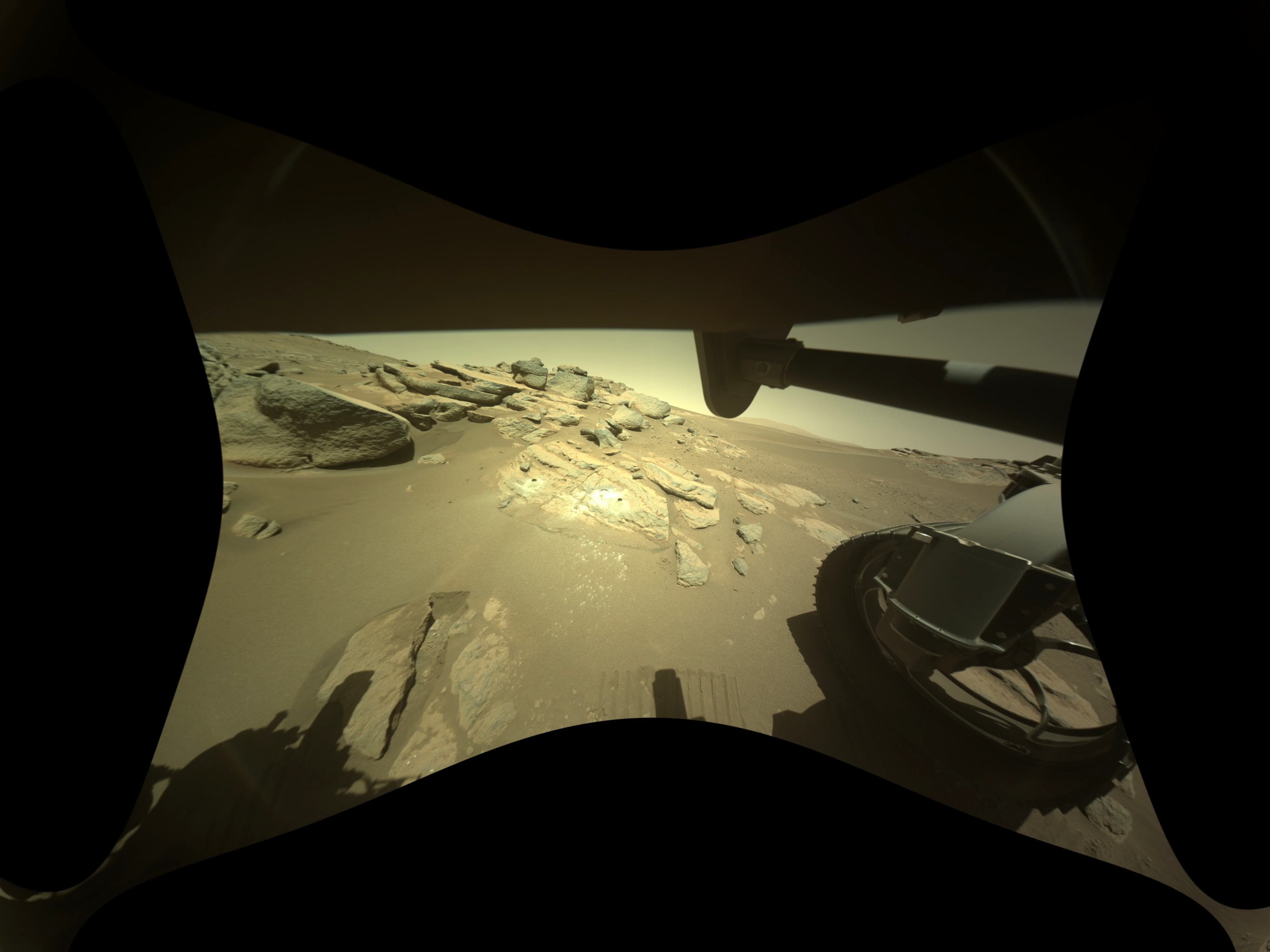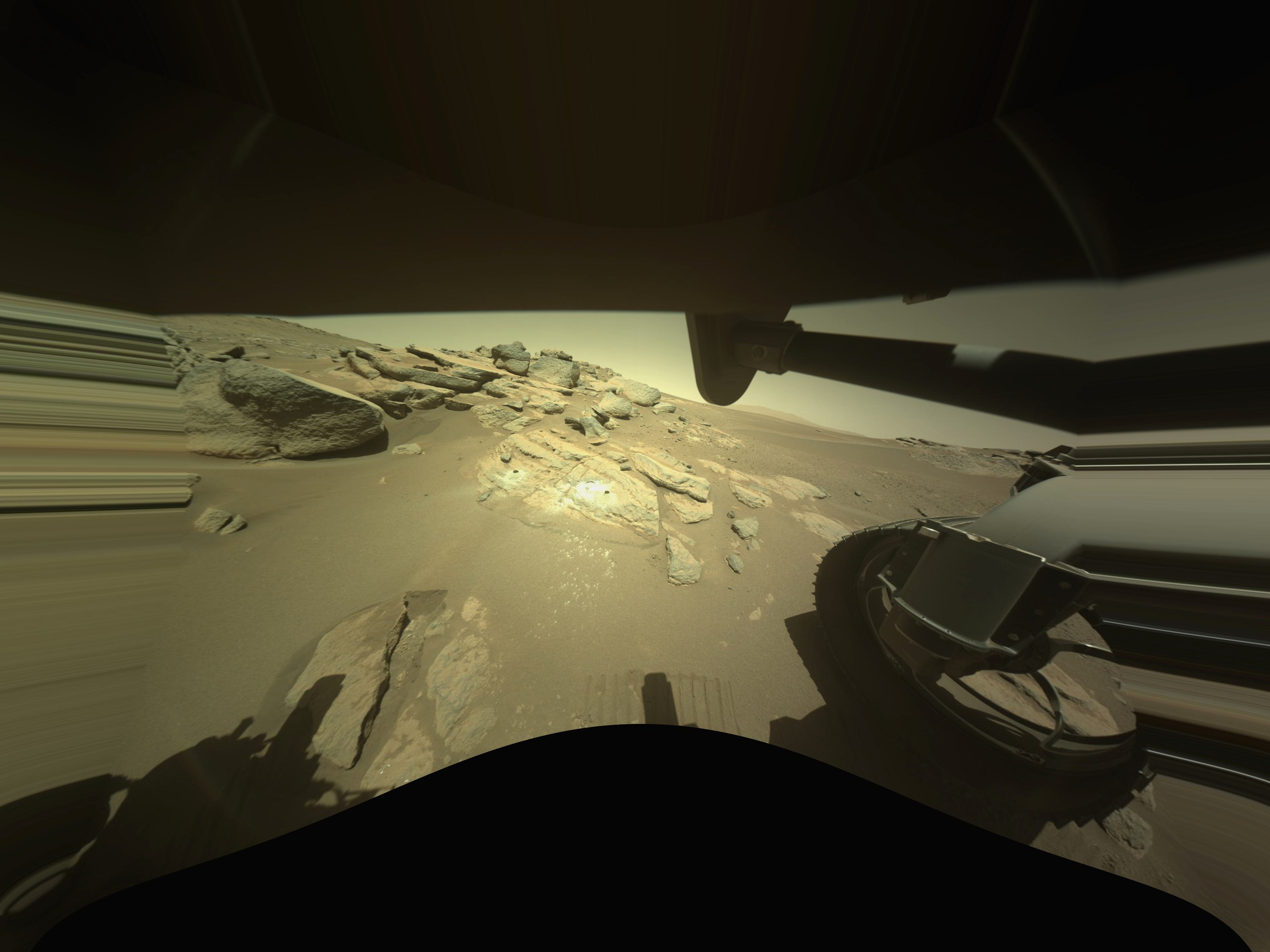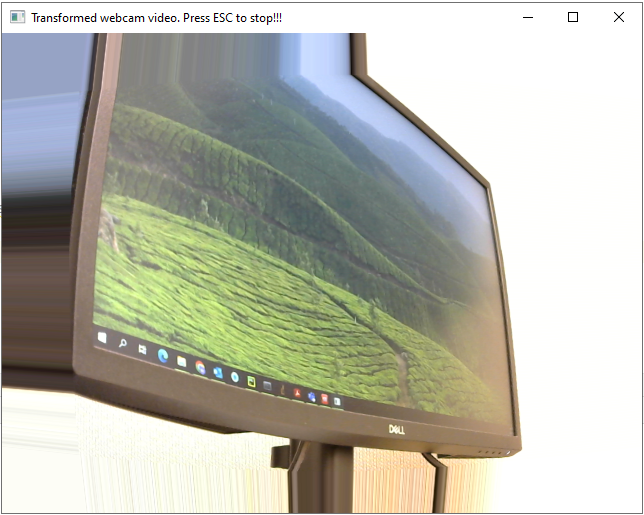# 3.3.9. Useful tips

1. Apply distortion correction to a color image. Given distortion coefficients from the calibration process shown in previous demos, we have to apply unwarping to each channel of the image as follows:

```import numpy as np
import discorpy.proc.processing as proc
import discorpy.post.postprocessing as post

file_path = "../../data/percy_cam/F_R_hazcam.png"
output_base = "E:/output/unwarping/"

# Define a function for convenient use.
def unwarp_color_image(img, xcenter, ycenter, list_bfact, mode='reflect'):
if len(img.shape) != 3:
raise ValueError("Check if input is a color image!!!")
img_corrected = []
for i in range(img.shape[-1]):
img_corrected.append(post.unwarp_image_backward(img[:, :, i], xcenter,
ycenter, list_bfact, mode=mode))
img_corrected = np.moveaxis(np.asarray(img_corrected), 0, 2)
return img_corrected

# Suppose that we get xcenter, ycenter, list_bfact (backward mode) to this point
img_corrected = unwarp_color_image(img, xcenter, ycenter, list_bfact)
io.save_image(output_base + "/corrected_F_R_hazcam.png", img_corrected)
```
2. Find the coordinates of a point in one space given its corresponding coordinates in another space. Suppose that we get distortion coefficients of the backward model; given a point in a distorted image, we want to find its position in the undistorted image. This can be done as the following:

```import numpy as np
import discorpy.proc.processing as proc
import discorpy.post.postprocessing as post

# Suppose that we get coefficients of the backward model (xcenter, yxenter, list_bfact).
# We need to find the forward transformation using the given backward model.
(height, width) = img.shape
ref_points = [[i - ycenter, j - xcenter] for i in np.linspace(0, height, 40) for j in
np.linspace(0, width, 40)]
list_ffact = proc.transform_coef_backward_and_forward(list_bfact, ref_points=ref_points)

# Define the function to calculate corresponding points between distorted and undistorted space
# This function can be used both ways:
# In: distorted point, forward model :-> Out: undistorted point
# In: undistorted point, backward model :-> Out: distorted point
def find_point_to_point(points, xcenter, ycenter, list_fact):
"""
points : (row_index, column_index) of the point.
"""
xi, yi = points - xcenter, points - ycenter
ri = np.sqrt(xi * xi + yi * yi)
factor = np.float64(np.sum(list_fact * np.power(ri, np.arange(len(list_fact)))))
xo = xcenter + factor * xi
yo = ycenter + factor * yi
return xo, yo

# Find top-left point in the undistorted space given top-left point in the distorted space.
xu_top_left, yu_top_left = find_point_to_point((0, 0), xcenter, ycenter, list_ffact)
# Find bottom-right point in the undistorted space given bottom-right point in the distorted space.
xu_bot_right, yu_bot_right = find_point_to_point((height - 1, width - 1), xcenter, ycenter,
list_ffact)
```
3. Maintain the original field of view of an image. In correction for the barrel distortion, some parts of the image edges will be cropped. However, there are two possible methods to incorporate these areas back into the undistorted image:

• Apply padding to the original image before unwarping. The appropriate amount of padding can be determined using the results from the example mentioned above. Note that we have to update the center of distortion corresponding to the padding.

```# Calculate padding width for each side.

list_bfact, mode='constant')
```• Rescale the distortion coefficients and resize the original image correspondingly.

```import scipy.ndimage as ndi

zoom = 2.0
list_bfact1 = zoom * list_bfact
xcenter1 = xcenter * zoom
ycenter1 = ycenter * zoom
img_corrected = []
for i in range(img.shape[-1]):
img_tmp = ndi.zoom(img[:, :, i], zoom)
img_tmp = post.unwarp_image_backward(img_tmp, xcenter1, ycenter1, list_bfact1)
img_corrected.append(img_tmp)
img_corrected = np.moveaxis(np.asarray(img_corrected), 0, 2)
io.save_image(output_base + "/F_R_hazcam_unwarped_zoom.jpg", img_corrected)
```1. To unwarp live images from a webcam or camera, it is recommended to use the remap function provided by OpenCV library due to its high performance. The following is a demonstration of its usage. However, it should be noted that for packaging purpose, Discorpy does not include this function in its API. Instead, map_coordinate function in Scipy is utilized.

```import os
import numpy as np
import cv2

def mapping_cv2(mat, xmat, ymat, method=cv2.INTER_LINEAR,
border=cv2.BORDER_CONSTANT):
"""
Apply a geometric transformation to a 2D array using Opencv.

Parameters
----------
mat : array_like.
Input image. Can be a color image.
xmat : array_like
2D array of the x-coordinates. Origin is at the left of the image.
ymat : array_like
2D array of the y-coordinates. Origin is at the top of the image.
method : obj
To select interpolation method. Note to use the prefix: cv2.<method>\\
https://docs.opencv.org/3.4/da/d54/group__imgproc__transform.html
border : obj
To select method for boundary handling. Note to use the prefix: cv2.<method> \\
https://docs.opencv.org/3.4/d2/de8/group__core__array.html#ga209f2f4869e304c82d07739337eae7c5

Returns
-------
array_like
"""
mat = cv2.remap(mat, xmat, ymat, interpolation=method, borderMode=border)
return mat

def unwarp_image_backward_cv2(mat, xcenter, ycenter, list_fact,
method=cv2.INTER_LINEAR,
border=cv2.BORDER_CONSTANT):
"""
Unwarp an image using the backward model with the Opencv remap method for
fast performance.

Parameters
----------
mat : array_like
Input image. Can be a color image.
xcenter : float
Center of distortion in x-direction.
ycenter : float
Center of distortion in y-direction.
list_fact : list of float
Polynomial coefficients of the backward model.
method : obj
To select interpolation method. Note to use the prefix: cv2.<method>\\
https://docs.opencv.org/3.4/da/d54/group__imgproc__transform.html
border : obj
To select method for boundary handling. Note to use the prefix: cv2.<method> \\
https://docs.opencv.org/3.4/d2/de8/group__core__array.html#ga209f2f4869e304c82d07739337eae7c5

Returns
-------
array_like
2D array. Distortion-corrected image.
"""
(height, width) = mat.shape[:2]
xu_list = np.arange(width) - xcenter
yu_list = np.arange(height) - ycenter
xu_mat, yu_mat = np.meshgrid(xu_list, yu_list)
ru_mat = np.sqrt(xu_mat**2 + yu_mat**2)
fact_mat = np.sum(
np.asarray([factor * ru_mat**i for i,
factor in enumerate(list_fact)]), axis=0)
xd_mat = np.float32(np.clip(xcenter + fact_mat * xu_mat, 0, width - 1))
yd_mat = np.float32(np.clip(ycenter + fact_mat * yu_mat, 0, height - 1))
mat = mapping_cv2(mat, xd_mat, yd_mat, method=method, border=border)
return mat

def unwarp_video_cv2(cam_obj, xcenter, ycenter, list_fact,
method=cv2.INTER_LINEAR, border=cv2.BORDER_CONSTANT):
"""
Unwarp frames from Opencv video object using the backward model.

Parameters
----------
cam_obj : obj
Opencv camera object. e.g. cv2.VideoCapture(0)
xcenter : float
Center of distortion in x-direction.
ycenter : float
Center of distortion in y-direction.
list_fact : list of float
Polynomial coefficients of the backward model.
method : obj
To select interpolation method. Note to use the prefix: cv2.<method>\\
https://docs.opencv.org/3.4/da/d54/group__imgproc__transform.html
border : obj
To select method for boundary handling. Note to use the prefix: cv2.<method> \\
https://docs.opencv.org/3.4/d2/de8/group__core__array.html#ga209f2f4869e304c82d07739337eae7c5

Returns
-------
Generator
"""
width = int(cam_obj.get(cv2.CAP_PROP_FRAME_WIDTH))
height = int(cam_obj.get(cv2.CAP_PROP_FRAME_HEIGHT))
xu_list = np.arange(width) - xcenter
yu_list = np.arange(height) - ycenter
xu_mat, yu_mat = np.meshgrid(xu_list, yu_list)
ru_mat = np.sqrt(xu_mat**2 + yu_mat**2)
fact_mat = np.sum(
np.asarray([factor * ru_mat**i for i,
factor in enumerate(list_fact)]), axis=0)
xd_mat = np.float32(np.clip(xcenter + fact_mat * xu_mat, 0, width - 1))
yd_mat = np.float32(np.clip(ycenter + fact_mat * yu_mat, 0, height - 1))
while True:
if check:
uframe = mapping_cv2(frame, xd_mat, yd_mat, method=method,
border=border)
cv2.imshow('Transformed webcam video. Press ESC to stop!!!', uframe)
c = cv2.waitKey(1)
if c == 27:
break
cam_obj.release()
cv2.destroyAllWindows()

def save_color_image_cv2(file_path, image):
"""
Convenient method for saving color image which creates a folder if it
doesn't exist.
"""
file_base = os.path.dirname(file_path)
if not os.path.exists(file_base):
try:
os.makedirs(file_base)
except OSError:
raise ValueError("Can't create the folder: {}".format(file_path))
cv2.imwrite(file_path, image)

#---------------------------------------------------------------------------
# Demonstration of using above functions for unwarping images from a webcam.
#---------------------------------------------------------------------------

# Open the webcam
cam_obj = cv2.VideoCapture(0)
# Get height and width
width = int(cam_obj.get(cv2.CAP_PROP_FRAME_WIDTH))
height = int(cam_obj.get(cv2.CAP_PROP_FRAME_HEIGHT))

# For demonstration, assuming that we get the following coefficients.
xcenter = width / 2.0
ycenter = height / 2.0
list_power = np.asarray([1.0, 10**(-3), 10**(-7)])
list_coef = np.asarray([1.0, 1.0, 1.0])
list_fact = list_power * list_coef

# Get a single image from the camera, apply correction, and save the result.